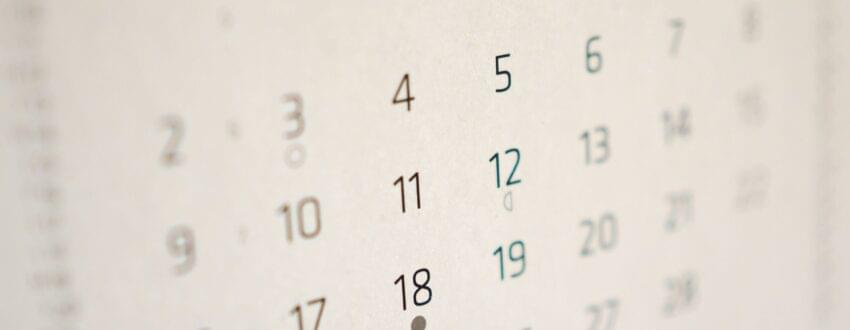NB: Make sure you do a back up of your theme, files and database before attempting the tutorialsEasyEstimated reading time : 2 minutes, 35 seconds - 249 words

With this tutorial, we will show you how to calculate the number of days between two dates. And with our example, We will also see an example of mathematical calculation with a Formidable form shortcode.

Let’s create a shortcode to calculate the number of days.

## Calculate the number of days between two dates

```function themespress_numberdays_shortcode() {
\$now = time(); // Current time
\$your_date = strtotime('2022-01-23'); // The first date
\$datediff = abs(\$now - \$your_date); // Gives absolute Value
return floor(\$datediff/(60*60*24)); // Returns the lowest value by rounding down value
}
// register shortcode

Our date ‘2022-01-23′ will be the start date and the variable \$now represents the current time. Afterwards, we need to calculate the number of days between two dates.

We can do calculations and statistics with the following Formidable Form shortcode:

`[frm-math][/frm-math]`

With this mathematical shortcode, we will insert the data to calculate. For example, we want to calculate the daily grocery expenses between two dates.

For example, you can create a Formidable Form to include grocery expenses.

Form fields:

1 – A hidden field with the default value of the date where the entry was created [date]

2 – Select Options field with various grocery stores (Ex: Costco, Walmart, etc…)

3 – A number field with the amount

After adding some data, you can proceed with the calculation with two shortcodes:

1 – We want to obtain the amount total (with entry ID) that will be the ID of my amount field.

```// Remplacez 321 par l'ID de votre entrée montant.
[frm-stats id=321 type=total]```

2 – The shortcode for which calculates the number of days with the function created above. [numberdays].

```// Fonction de notre calcul du nombre de jours.
[numberdays]```

Now, calculate the results on one of your pages:

```// Remplacez 321 par l'ID de votre entrée montant.
[frm-math][frm-stats id=321 type=total] / [numberdays][/frm-math]```

With Formidable Form, you can generate charts to display your results.

```// Remplacez 320 par l'ID du champ select options des supermarchés
[frm-graph fields="320" type="column" data_type="total"]```